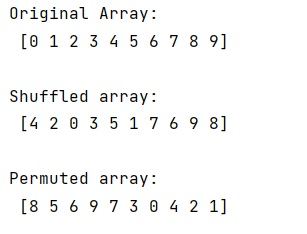# shuffle vs permute numpy

Learn about the difference between NumPy's shuffle method and permute method.
Submitted by Pranit Sharma, on January 11, 2023

NumPy is an abbreviated form of Numerical Python. It is used for different types of scientific operations in python. Numpy is a vast library in python which is used for almost every kind of scientific or mathematical operation. It is itself an array which is a collection of various methods and functions for processing the arrays.

## NumPy - shuffle vs permute Methods

Numpy's random.shuffle() method modifies a sequence in-place by shuffling its contents. This function only shuffles the array along the first axis of a multi-dimensional array. The order of sub-arrays is changed but their contents remain the same.

Numpy's random.permute() randomly permute a sequence, or return a permuted range. If x is a multi-dimensional array, it is only shuffled along its first index.

Basically, np.random.permutation() has two differences from np.random.shuffle():

• If passed an array, it will return a shuffled copy of the array;
np.random.shuffle() shuffles the array in place
• if passed an integer, it will return a shuffled range i.e. np.random.shuffle(np.arange(n))

Let us understand with the help of an example,

## Python code to demonstrate the example of NumPy's shuffle vs permute methods

```# Import numpy
import numpy as np

# Creating an array
arr = np.arange(10)

# Display original array
print("Original Array:\n",arr,"\n")

# Shuffling array
np.random.shuffle(arr)

# Display result
print("Shuffled array:\n",arr,"\n")

# using permutation method
res = np.random.permutation(arr)

# Display result
print("Permuted array:\n",res)
```

Output:What's New (MCQs)

Top Interview Coding Problems/Challenges!

IncludeHelp's Blogs

Languages: » C » C++ » C++ STL » Java » Data Structure » C#.Net » Android » Kotlin » SQL
Web Technologies: » PHP » Python » JavaScript » CSS » Ajax » Node.js » Web programming/HTML
Solved programs: » C » C++ » DS » Java » C#
Aptitude que. & ans.: » C » C++ » Java » DBMS
Interview que. & ans.: » C » Embedded C » Java » SEO » HR
CS Subjects: » CS Basics » O.S. » Networks » DBMS » Embedded Systems » Cloud Computing
» Machine learning » CS Organizations » Linux » DOS
More: » Articles » Puzzles » News/Updates